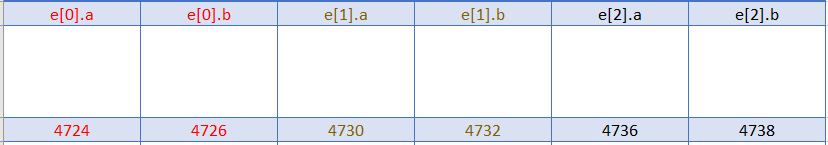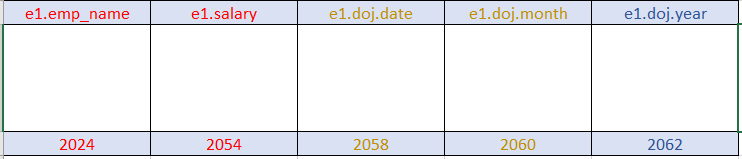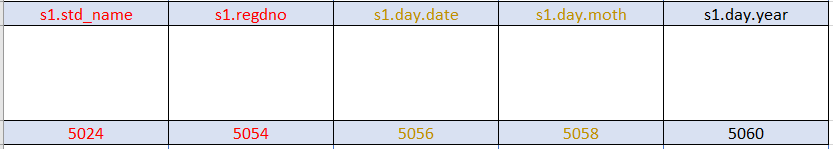In this tutorial, we are going to learn about accessing members of the structure.

## Access the members of a Struct with an arrow (->) operator:

pointer pointing to a structure is known as a structure pointer. If a pointer to the structure variable is declared, then arrow (->) notation is used to access the members of the structure.

Remember that on the left-hand side of the dot operator there must always be a structure variable, whereas on the left-hand side of the arrow operator there must always be a pointer to a structure.

To access a member of a structure using the arrow (->) operator, the syntax is:

``structure_pointer_variable -> member``

Let us consider an example:

``````struct student {
int roll_number;
int total_marks;
float attendance_percentage;
};
struct student s = {10, 95, 98.45};
struct student *p;
p = &s;
printf("%d %d %f\n", p->roll_number, p->total_marks, p->attendance_percentage);``````

In the above example, p is a pointer to the structure student, so it can store the address of a structure variable of the same type.

Here, p->roll_number refers the value of s.roll_number, p->total_marks refers the value of s.total_marks and p->attendance_percentage refers the value of s.attendance_percentage.

Let us consider an example below:

``````#include <stdio.h>
void main() {
struct book {
char name;
int pages;
float price;
};
struct book b = {"How to enjoy life", 542, 100.23};
struct book *p;
p = &b;
printf("Details of the book are:\nName : %s\nNumber of Pages : %d\nPrice of the book : %f\n", p->name, p->pages, p->price);
}``````

Output:

``````Details of the book are:
Name : How to enjoy life
Number of Pages : 542
Price of the book : 100.230003``````

## An array of Structures:

When the programmer wants to define more structure variables, it is better to practice defining an array of structures rather than individual structure variables.

The creation of an array of structures is the same as the creation of integer arraysfloat arrays, and so on.

The syntax of defining an array of structure is:

``struct tag_name structure_variable[size]; //the size specifies the number of structure variables to be created``

Below is an example of an array of structure:

``````struct example {
int a;
float b;
};
struct example e;``````

In the above example, the definition of a structure will create an array of structures with 3 structure variables of type structure example. An array of structure variables should get a memory of continuous blocks and they can be accessed sequentially.Let us consider an example:

``````#include <stdio.h>
void main() {
struct student {
int roll_number;
int marks;
};
struct student s;
int i,n;
printf("Enter number of students :");
scanf("%d", &n);
for(i = 0; i < n; i++) {
printf("Enter the roll number : ");
scanf("%d", &s[i].roll_number);
printf("Enter the marks in C language : ");
scanf("%d", &s[i].marks);
}
printf("\nGiven details are :-\n");
for(i = 0; i < n; i++) {
printf("Roll number : %d\n Marks in C language are : %d\n", s[i].roll_number, s[i].marks);
}
}
``````

Output:

``````Enter number of students :5
Enter the roll number : 1
Enter the marks in C language : 23
Enter the roll number : 2
Enter the marks in C language : 24
Enter the roll number : 3
Enter the marks in C language : 25
Enter the roll number : 4
Enter the marks in C language : 19
Enter the roll number : 5
Enter the marks in C language : 20

Given details are :-
Roll number : 1
Marks in C language are : 23
Roll number : 2
Marks in C language are : 24
Roll number : 3
Marks in C language are : 25
Roll number : 4
Marks in C language are : 19
Roll number : 5
Marks in C language are : 20``````

## Nested Structures:

There are two ways to define nested structures in C:

1. Define one structure variable within the declaration of another structure.
2. Declare one structure within the declaration of another structure.

Below is an example of defining one structure variable within another structure:

``````struct date {
int date;
int month;
int year;
};

struct employee {
char emp_name;
float salary;
struct date doj;
} e1;

struct student {
char std_name;
int regdno;
struct date day;
}s1;``````

In the above example, date is one user-defined data type that is declared outside of all structures. The structure variables of date are defined within two structures employee and student.

The structure variable e1 is defined for the type structure employee, which allocates the memory to each and every field including the structure variable doj of date.The structure variable s1 is defined for the type structure student, which allocates the memory to each and every field including the structure variable day of date.When the structure date is declared in the global scope, the structure variables of date can be defined anywhere in the program. We can access the member of nested structure as given below:

Syntax:

``````    Outer_Structure.Nested_Structure.member
Example:
e1.doj.date
s1.day.month``````

Let us consider an example:

``````#include <stdio.h>
struct date {
int date;
int month;
int year;
};
struct employee {
char ename;
float salary;
struct date doj;
};
void main() {
struct employee emp1 = {"Ankith", 1200.32, {28, 1, 2000}};
printf("Employee Name    : %s\n", emp1.ename);
printf("Employee Salary  : %f\n", emp1.salary);
printf("Employee DOJ     : %d/%d/%d\n", emp1.doj.date, emp1.doj.month, emp1.doj.year);
}``````

Output:

``````Employee Name    : Ankith
Employee Salary  : 1200.319946
Employee DOJ     : 28/1/2000``````

## Accessing structures through functions:

Like an ordinary variable, a structure variable can also be passed to a function. Users may either pass individual structure elements or the entire structure at once.

If a structure variable is passed by value, changes made to the structure variable inside the function definition do not reflect in the originally passed structure variable.

If a structure variable is passed by address, changes made to the structure variable inside function definition reflect in the originally passed structure variable.

If a programmer wants to pass a structure variable to the function, first the programmer has to define the structure in the global section then only the function can access that structure variable.

``````#include <stdio.h>
struct book {
char name;
float price;
int pages;
};
void display(struct book);
void main() {
struct book b = {"How to enjoy life", 452.213, 500};
display(b);
}
void display(struct book b) {
printf("Given book details are \nName : %s\nNumber of pages : %d\nPrice of the book : %f\n", b.name, b.pages, b.price);
}``````

Output:

``````Given book details are
Name : How to enjoy life
Number of pages : 500
Price of the book : 452.213013``````

## Understanding structures with pointers:

A pointer pointing to a structure is known as a structure pointer. A pointer to the structure can be defined with an asterisk (*) symbol.

Remember that on the left-hand side of the dot (.) operator there must always be a structure variable, whereas on the left-hand side of the arrow (->) operator there must be always a pointer to the structure.

A pointer variable to the structure can hold the address of a structure variable or address of a heap memory allocated at run-time for the structure type.

``````#include <stdio.h>
struct student {
float avg;
int roll;
};
void display(struct student *);
void main() {
struct student s;
display(&s);
}
printf("Enter the average of the student : ");
scanf("%f", &k->avg);
printf("Enter the roll number of the student : ");
scanf("%d", &k->roll);
}
void display(struct student *k) {
printf("Given details are :\nRoll Number of the student : %d\nAverage mark of the student : %f\n", k->roll, k->avg);
}
``````

Output:

``````Enter the average of the student : 98.4
Enter the roll number of the student : 10
Given details are :
Roll Number of the student : 10
Average mark of the student : 98.400002``````

Happy Learning 🙂